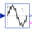# TotalHarmonicDistortion

Calculation of total harmonic distortion of voltage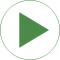# Information

This information is part of the Modelica Standard Library maintained by the Modelica Association.

This example compares the result of the total harmonic distortion (THD) with respect to the fundamental wave and with respect to the total root mean square (RMS). In this simulation model a non-sinusoidal voltage wave form is created by the superposition two voltage waves:

• Fundamental wave with RMS voltage `V1` and frequency `f1`
• Third harmonic wave with RMS voltage `V3` and frequency `3*f1`

This simulation model compares numerically determined THD values with results, obtained by theoretical calculations:

• Compare the numerically determined THD value `thd1.y` and the theoretical value `THD1`, both with respect to the fundamental wave; also plot `thd1.valid`
• Compare the numerically determined THD value `thdRMS.y` and the theoretical value `THDrms`, both with respect to the RMS value; also plot `thdRMS.valid`

# Parameters (5)

f1 Value: 50 Type: Frequency (Hz) Description: Fundamental wave frequency Value: 100 Type: Voltage (V) Description: Fundamental wave RMS voltage Value: 20 Type: Voltage (V) Description: Third harmonic wave RMS voltage Value: V3 / V1 Type: Real Description: Theoretically obtained THD with respect to fundamental wave Value: V3 / sqrt(V1 ^ 2 + V3 ^ 2) Type: Real Description: Theoretically obtained THD with respect to RMS

# Components (6)

ground sineVoltage3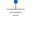Type: Ground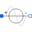Type: SineVoltageType: SineVoltage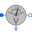Type: VoltageSensor